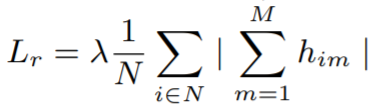# Regularizers¶

Regularizers are applied to weights and embeddings without the need for labels or tuples.

Here is an example of a weight regularizer being passed to a loss function.

``````from pytorch_metric_learning import losses, regularizers
R = regularizers.RegularFaceRegularizer()
loss = losses.ArcFaceLoss(margin=30, num_classes=100, embedding_size=128, weight_regularizer=R)
``````

## BaseRegularizer¶

``````regularizers.BaseWeightRegularizer(collect_stats = False,
reducer = None,
distance = None)
``````

An object that extends this class can be passed as the `embedding_regularizer` into any loss function. It can also be passed as the `weight_regularizer` into any class that extends WeightRegularizerMixin.

Parameters

• collect_stats: If True, will collect various statistics that may be useful to analyze during experiments. If False, these computations will be skipped. Want to make `True` the default? Set the global COLLECT_STATS flag.
• reducer: A reducer object. If None, then the default reducer will be used.
• distance: A distance object. If None, then the default distance will be used.

Default distance:

Default reducer:

## CenterInvariantRegularizer¶

Deep Face Recognition with Center Invariant Loss

This encourages unnormalized embeddings or weights to all have the same Lp norm.

``````regularizers.CenterInvariantRegularizer(**kwargs)
``````

Default distance:

Default reducer:

## LpRegularizer¶

This encourages embeddings/weights to have a small Lp norm.

``````regularizers.LpRegularizer(p=2, power=1, **kwargs)
``````

Parameters

• p: The type of norm. For example, `p=1` is the Manhattan distance, and `p=2` is Euclidean distance.

Default distance:

• This regularizer does not use a distance object, so setting this parameter will have no effect.

Default reducer:

## RegularFaceRegularizer¶

RegularFace: Deep Face Recognition via Exclusive Regularization

This should be applied as a weight regularizer. It penalizes class vectors that are very close together.

``````regularizers.RegularFaceRegularizer(**kwargs)
``````

Default distance:

Default reducer:

## SparseCentersRegularizer¶

SoftTriple Loss: Deep Metric Learning Without Triplet Sampling

This should be applied as a weight regularizer. It encourages multiple class centers to "merge", i.e. group together.

``````regularizers.SparseCentersRegularizer(num_classes, centers_per_class, **kwargs)
``````

Parameters

• num_classes: The number of classes in your training dataset.
• centers_per_class: The number of rows in the weight matrix that correspond to 1 class.

Default distance:

Default reducer:

## ZeroMeanRegularizer¶

Signal-to-Noise Ratio: A Robust Distance Metric for Deep Metric Learning

``````regularizers.ZeroMeanRegularizer(**kwargs)
``````

Equation

In this equation, `N` is the batch size, `M` is the size of each embedding.Default distance:

• This regularizer does not use a distance object, so setting this parameter will have no effect.

Default reducer: